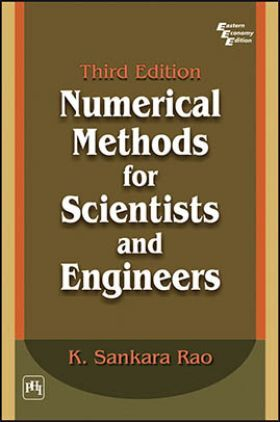# Numerical Methods For Scientists And Engineers

( 56 )
Sold ( 60 times )
17715 Views

This product is currently not available.

Save extra with 1 Offers

Get ₹ 50

Instant Cashback on the purchase of ₹ 400 or above

Product Specifications

 Publisher PHI Learning All Engineering Mathematics books by PHI Learning ISBN 9788120332171 Author: SANKARA RAO, K. Number of Pages 368 Edition Third Edition Available Available in all digital devicesAbout The Book Numerical Methods For Scientists And Engineers

Book Summary:

Primarily written as a textbook, this third edition provides a complete course on numerical methods for undergraduate students in all branches of engineering, postgraduate students in mathematics and physics, and students pursuing courses in Master of Computer Applications (MCA). Besides students, those appearing for competitive examinations, research scholars and professionals engaged in numerical computations, will treasure this edition for its in-depth analysis, systematic treatment and clarity of approach.

The third edition has been updated with new material comprising new methods and concepts and additional chapters on Boundary Value Problems and Approximation of Functions. It introduces the basics in computing, stresses on errors in computation, discusses various direct and iterative methods for solving algebraic and transcendental equations and a method for solving a system of nonlinear equations, linear system of equations, matrix inversion and computation of eigenvalues and eigenvectors of a matrix.

The book provides a detailed discussion on curve fitting, interpolation and cubic spline interpolation, numerical differentiation and integration. It also presents, various single step and predictorcorrector methods for solving ordinary differential equations, finite difference methods for solving partial differential equations with the concepts of truncation error and stability. Finally, it concludes with a treatment of numerical methods for solving boundary value problems, least squares, Chebyshev, Pade polynomial approximations and Fourier series approximation to a real continuous function.

KEY FEATURES

Provides altogether about 300 examples, of which about 125 are worked-out examples.

Gives detailed hints and solutions to examples under Exercises.

Preface
Preface to the Second Edition
1. Basics in Computing
2. Solution of Algebraic and Transcendental Equations
3. Solution of Linear System of Equations and Matrix Inversion
4. Eigenvalue Problems
5. Curve Fitting
6. Interpolation
7. Numerical Differentiation and Integration
8. Ordinary Differential Equations
9. Parabolic Partial Differential Equations
10. Elliptic Partial Differential Equations
11. Hyperbolic Partial Differential Equations
12. Boundary Value Problems
13. Approximation of Functions
Appendix
Bibliography